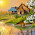### Electric Potential and Potential Difference

When a mass is raised above the ground level, work is done against the force of gravity. This work done is stored in the mass as a potential energy (mgh). Hence, due to such, potential energy it is said that the mass, when raised above the ground level has a gravitational potential such. Potential of mass depends upon the position of the mass with respect to the ground.
An electric charge gives rise to an electric field around it, analogous to gravitational field around the earth. If any charge is introduced in this field, it gets attracted or repelled, depending on the nature of the charge. At the time of movement of this charge, work is done against or by the force acting on the charge due to the electric field. This depends on the position of the charge in the electric field and is analogous to the proportional of mass due to gravitation field, when lifted upwards.
Now, consider a small isolated positive charge 'q' placed at infinity with respect to another isolated positive charge 'q' as shown in the Fig 1. theoretically, the electric field of charge 'q' extends upto infinity but has a zero influence at infinity, where 'q' is placed. When charge 'q' is moved towards 'Q', work is done against the force of repulsion between these two like charges.

Due to this work done, when charge 'q' reaches position A, it acquires a potential energy. If charge 'q' is released, due to force of repulsion, it will go back to infinity i.e. position of zero potential. So, at point A, charge 'q' has some potential exactly equal to work done in bringing it from infinity to the point A, called electric potential.
It can be defined as the work done in joules, in moving a unit positive charge from infinity (position of zero potential) to te point against the electric field.
It is denoted by symbol V and is measured in joule per coulomb or volt.

Definition of 1 volt :
The electric potential at a point in an electric field is said to be one volt when the work done in bringing a unit positive charge from infinity to that point from infinity against the electric field is one joule.

1.1 Potential Difference
Consider two points A and B in an electric field as shown in Fig 2. The positive charge '+q' is moved from point A to B in an electric field. At point A, charge acquires certain electric potential say VA. Some additional work is done in bringing it to point B, it has an electric potential say VB.

Note: The difference between these two potentials per unit positive charge is called potential difference.
So, the potential difference between the two points in an electric field is defined as the work done in moving a unit positive charge from the point of lower potential to the higher potential.

1.2 Expressions for Potential and Potential Difference
Consider a positive charge Q placed in a medium of relative permittivity. Consider a point P at a distance r from the charge Q. Now, a unit positive charge of 1 c is placed at point P, there will exist a force of repulsion between the two charges. This is shown in the Fig 3.

The force of repulsion is given by,

Now electric field intensity at point P is the ratio of force to charge at point P. But charge at P is unit charge,

Now, move the unit charge at point P towards charge Q against the force of repulsion
Work Done:
Let the distance moved by charge at P towards Q be dr and for this work is done against force of repulsion, given by,

The negative sign indicates that the work is done against the force of repulsion.
Now, to find electrical potential at point P, consider that the unit positive charge is moved from infinity to the point P. hence, total wok done in moving unit positive charge is from infinity to point P can be obtained by integrating dW as,

Note: But this total work done is nothing but potential at point P.

Thus, as r increases, potential decreases till it becomes zero at infinity.
Potential difference :
Consider point A at a distance d1 from charge Q. Hence, potential of point A is given by,

While the potential of point B which is at distance d2 from the charge Q is,
Hence, the potential difference between the points A and B is given by,

We know that field intensity at a distance 'd' due to charge Q is given by,

While the potential of the same point is given by,

Substituting V in expression for E, we can write,

#### 1 comment:

1.hi dear its blog very nice and helpful thanks from inverter